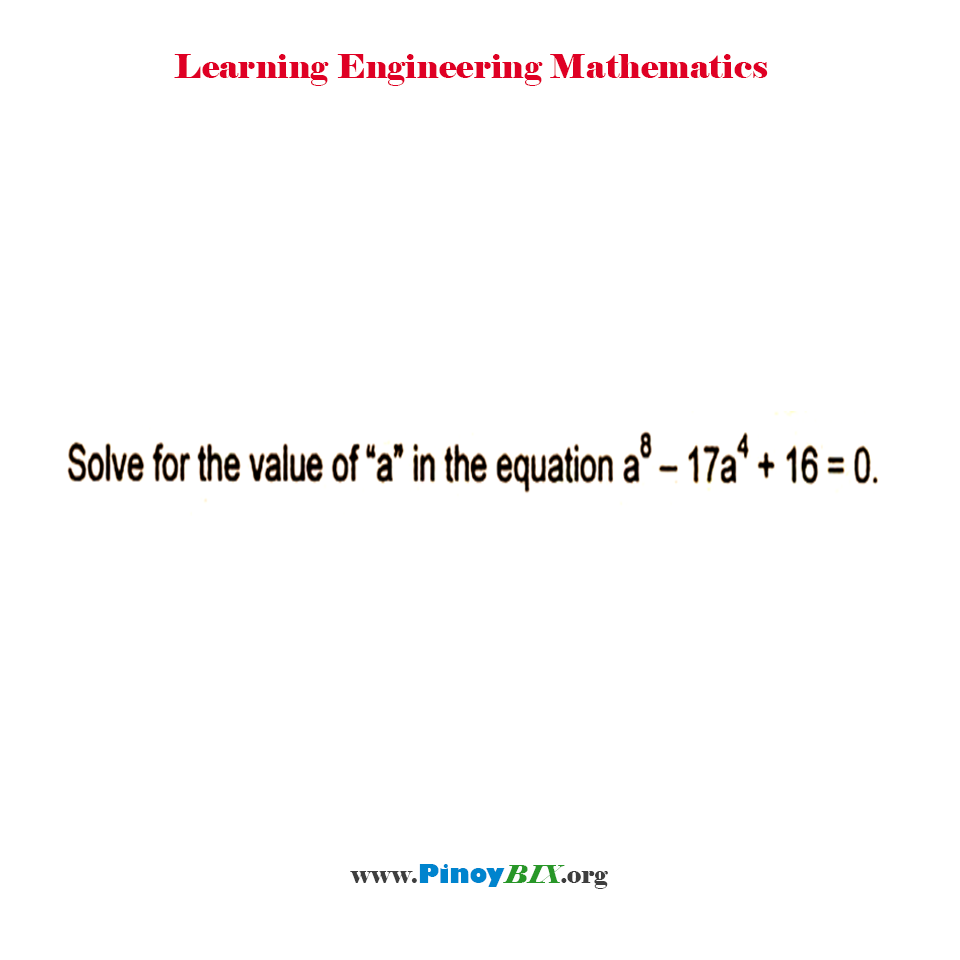# Solution: Solve for the value of “a” in the equation a^8–17a^4+16=0

(Last Updated On: January 21, 2020)#### Problem Statement: ECE Board April 1990

Solve for the value of “a” in the equation a^8–17a^4+16=0.

• A. ±2
• B. ±3
• C. ±4
• D. ±5

#### Problem Answer:

The value of “a” in the equation is ±2.

#### Latest Problem Solving in Quadratic Equation, Binomial Theorem and Logarithms

More Questions in: Quadratic Equation, Binomial Theorem and Logarithms

#### Online Questions and Answers in Quadratic Equation, Binomial Theorem and Logarithms

Please do Subscribe on YouTube!

P inoyBIX educates thousands of reviewers and students a day in preparation for their board examinations. Also provides professionals with materials for their lectures and practice exams. Help me go forward with the same spirit.

“Will you subscribe today via YOUTUBE?”

Subscribe